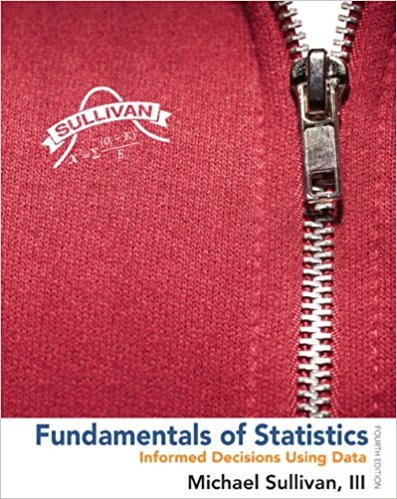×
Get Full Access to Fundamentals Of Statistics - 4 Edition - Chapter 6.2 - Problem 39ayu
Get Full Access to Fundamentals Of Statistics - 4 Edition - Chapter 6.2 - Problem 39ayu

×

# Solved: Sneeze According to a study done by Nick Wilson ofISBN: 9780321838704 30

## Solution for problem 39AYU Chapter 6.2

Fundamentals of Statistics | 4th Edition

• Textbook Solutions
• 2901 Step-by-step solutions solved by professors and subject experts
• Get 24/7 help from StudySoup virtual teaching assistantsFundamentals of Statistics | 4th Edition

4 5 1 391 Reviews
17
4
Problem 39AYU

Sneeze  According to a study done by Nick Wilson of Otago University Wellington, the probability a randomly selected individual will not cover his or her mouth when sneezing is 0.267. Suppose you sit on a bench in a mall and observe people’s habits as they sneeze.

(a) What is the probability that among 10 randomly observed individuals exactly 4 do not cover their mouth when sneezing?

(b) What is the probability that among 10 randomly observed individuals fewer than 3 do not cover their mouth?

(c) Would you be surprised if, after observing 10 individuals, fewer than half covered their mouth when sneezing? Why?

Step-by-Step Solution:

Step 1 of 3

Given:

Sneeze According to a study done by Nick Wilson of Otago University Wellington, the probability a randomly selected individual will not cover his or her mouth when sneezing is 0.267. Suppose you sit on a bench in a mall and observe people’s habits as they sneeze.

a)

Let x be the number of person do not cover their mouth.

Let the probability a randomly selected individual will not cover his or her mouth when sneezing is 0.267.

P = 0.267

Let the sample size n = 10.

Let x follows binomial distribution with parameter np.

Here n = 10 and P = 0.267

The probability of a mass function of a binomial distribution is

P(X = x) =, for x = 0, 1, 2, . . . , n

Here we find the probability that among 10 randomly observed individuals exactly 4 do not cover their mouth when sneezing.

P(X = 4) =P(X = 4) = 0.165534

P(X = 4)0.1655

Therefore the probability exactly 4 do not cover their mouth when sneezing is 0.1655

In 100 trials of this experiment,we would expect about 2 trials  to trials in exactly 4  do not cover their mouth when sneezing.

Step 2 of 3

Step 3 of 3

##### ISBN: 9780321838704

This textbook survival guide was created for the textbook: Fundamentals of Statistics, edition: 4. This full solution covers the following key subjects: mouth, Individuals, sneezing, Randomly, cover. This expansive textbook survival guide covers 51 chapters, and 2146 solutions. The full step-by-step solution to problem: 39AYU from chapter: 6.2 was answered by , our top Statistics solution expert on 04/03/17, 08:02AM. Since the solution to 39AYU from 6.2 chapter was answered, more than 11513 students have viewed the full step-by-step answer. The answer to “?Sneeze According to a study done by Nick Wilson of Otago University Wellington, the probability a randomly selected individual will not cover his or her mouth when sneezing is 0.267. Suppose you sit on a bench in a mall and observe people’s habits as they sneeze.(a) What is the probability that among 10 randomly observed individuals exactly 4 do not cover their mouth when sneezing?(b) What is the probability that among 10 randomly observed individuals fewer than 3 do not cover their mouth?(c) Would you be surprised if, after observing 10 individuals, fewer than half covered their mouth when sneezing? Why?” is broken down into a number of easy to follow steps, and 101 words. Fundamentals of Statistics was written by and is associated to the ISBN: 9780321838704.

Unlock Textbook Solution